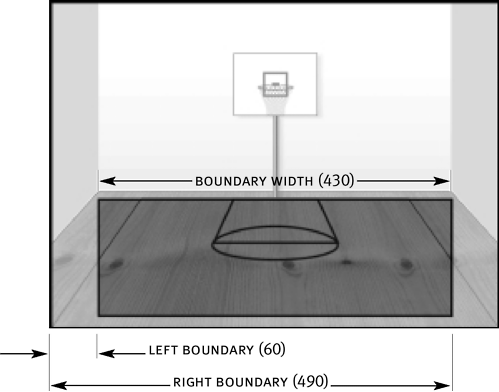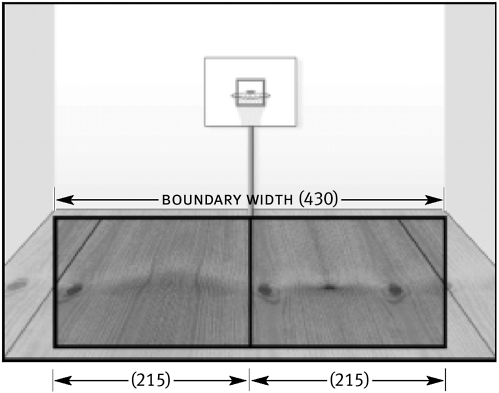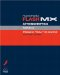# CONTROLLING PANNING

 While the volume of a sound gives a sense of distance, panning helps determine its left-right position. Like setting the volume of a Sound object, setting a Sound object's panning is straightforward, as the following example demonstrates: ` bounce.setPan (100); ` The above code will cause the bounce sound to play out of the right speaker only. You can set a Sound object's panning anywhere between 100 (left speaker only) and 100 (right speaker only), with a setting of 0 causing the sound to play equally out of both speakers.As with the setVolume() method, the setPan() method can also use the value of a variable to set a Sound object's panning more dynamically, as the following example demonstrates: ` bounce.setPan (myVariable); ` For our exercise, we'll use a variable to set the pan of our bounce Sound object. As in the previous exercise, this variable will contain a percentage value between 100 and 100 (encompassing the entire spectrum of panning values). We'll base the pan setting of the bounce Sound object on the horizontal distance of the mouse from the center point in either the left or right quadrant.To make this work, you must: Determine the horizontal size of our draggable boundary and then split it in two, essentially breaking the boundary into two "quadrants," left and right. Establish the position of the horizontal center. Determine the mouse's current horizontal position (at the exact center or in the left or right quadrant) each time it's moved. If the mouse is at the exact center point, the pan will be set to 0. If the mouse is to the left of the center point (in the left quadrant), pan will be set to a value between 100 and 0. This value represents the horizontal distance (percent-based) of the mouse from the center point in relation to the overall size of the quadrant. Likewise, if the mouse is to the right of the center point (in the right quadrant), pan will be set to a value between 0 and 100, which represents the horizontal distance (percent-based) of the mouse from the center point in relation to the overall size of the quadrant. Don't worry if you're confused. We've already discussed most of the principles for translating this logic into ActionScript; we just need to adapt them a bit. Open basketball4.fla in the Lesson16/Assets folder. If you wish, you can simply continue using the file you were working on at the end of the previous exercise. Select the basketball movie clip instance, then open the Actions panel. After the line of script that says boundaryHeight = bottomBoundary topBoundary , add the following line of script: ` boundaryWidth = rightBoundary leftBoundary; ` This creates a variable named boundaryWidth and assigns it a value based on what rightBoundary leftBoundary evaluates to. The lines of script directly above this indicate that rightBoundary = 490 and leftBoundary = 60 . Thus, this line of script written out would look like this: boundaryWidth = 490 60 or boundaryWidth = 430 This value represents the horizontal size of our boundary and will be used to determine the size of the left and right quadrants of the boundary.After the line of script that says boundaryWidth = rightBoundary leftBoundary, add the following line of script: ` quadrantSize = boundaryWidth / 2; ` This creates a variable named quadrantSize and assigns it a value based on what boundaryWidth / 2 evaluates to. At this point, boundaryWidth has a value of 430. Thus, this line of script written out would look like this: quadrantSize = 430 / 2 or quadrantSize = 215 You need to know the size of these quadrants to determine what percentage values to use to set the pan of our bounce Sound object.After the line of script that says quadrantSize = boundaryWidth / 2 , add the following line of script: ` centerPoint = rightBoundary quadrantSize; ` This creates a variable named centerPoint and assigns it a value based on what rightBoundary quadrantSize evaluates to. At this point, rightBoundary has a value of 490, and quandrantSize has a value of 215. Thus, this line of script written out would look like this: centerPoint = 490 215 or centerPoint = 275 This value denotes the horizontal location of the center point of the boundary the place where left and right quadrants meet. This variable plays a critical role in the panning process because it allows us to determine which quadrant the mouse is in and thus whether our bounce Sound object should be panned left or right. If the mouse's horizontal position (_root._xmouse ) is greater than centerPoint (275), we know that the mouse is in the right quadrant; if it's less than 275, we know the mouse is in the left quadrant. After the line of script that says quadrantSize = boundaryWidth / 2 , add the following lines of script: ` panAmount = ((_root._xmouse centerPoint) / quadrantSize) * 100; bounce.setPan (panAmount); ` In the first line the variable panAmount is created. The expression that sets the value of panAmount is based on the percent-generating equation we used to set volume. After the value of panAmount has been established, this variable is used to set the pan for the bounce Sound object, as shown on the last line above. The expression is set up to generate a value between 100 and 100 for panAmount . To help you understand the way this section of script works, we'll look at a couple of scenarios. First, let's assume that the mouse's horizontal position (_root._xmouse ) is 374 when the expression that sets the value of panAmount is evaluated. By plugging in the values for centerPoint (275) and quadrantSize (215), we can break down this expression in the following way: panAmount = ((374 275) / 215) * 100 or panAmount = (99 / 215) * 100 or panAmount = .4604 * 100 or panAmount = 46.04 Once the value of panAmount has been determined, the next line we added is executed. This line sets the pan of the bounce Sound object based on the value that the expression assigned to the panAmount variable. At this point, the value of panAmount is 46.04. Setting the pan to this amount will cause it to sound 46.04 percent louder in the right speaker than the left, indicating that the ball is on the right side of the basketball court. Visually, the ball will appear on the right side of the court as well, since the mouse's horizontal position (374) is greater than that of centerPoint (275), indicating that the mouse (and thus the basketball movie clip instance) is 99 pixels (374 275) to the right of the center point. Now let's look at one more scenario. Assume that the mouse's horizontal position is 158. Plugging all the necessary values into our expression, we can break it down as follows: panAmount = ((158 275) / 215) * 100 or panAmount = (-117 / 215) * 100 or panAmount = -.5442 * 100 or panAmount = -54.42 In this scenario, panAmount gets set to a negative number (-54.42). This is the result of subtracting 275 from 158, as is done at the beginning of the expression. Since 158 275 = -117 (a negative number), the expression evaluates to a negative value ideal since we need a negative value to pan our sound to the left. Once again, after the value of panAmount has been determined, the next line we added is executed. This line sets the pan of the bounce Sound object based on the value our expression assigned to the panAmount variable (-54.42). This causes the bounce to sound 54.42 percent louder in the left speaker than in the right, indicating that the ball is on the left side of the basketball court. Visually, the ball will appear on the left side of the court as well, since the mouse's horizontal position (158) is less than that of centerPoint (275), indicating that the mouse (and thus the basketball movie clip instance is 117 pixels (158 275) to the left of the center point. If the mouse's horizontal position is equal to that of centerPoint (275), the expression will set the value of panAmount to 0, causing sound to come out equally from both the left and right speakers. This in turn indicates that the mouse is in the center of the court. Since these two lines of script are nested within the mouseMove event handler as well as in the if statement that looks for movement within our boundary, the sound is panned each time the mouse is moved within the boundary. Choose Control > Test Movie to see how the movie operates. In the testing environment, move your mouse around the court. As you drag the ball, not only does the bounce's volume change, so does the location of the sound (moving from left to right). Close the testing environment to return to the authoring environment. Save the current file as basketball5.fla. You may want to keep this file open since we'll continue to build on it.Macromedia Flash MX ActionScripting: Advanced Training from the Source
ISBN: 0201770229
EAN: 2147483647
Year: 2002
Pages: 161

flylib.com © 2008-2017.
If you may any questions please contact us: flylib@qtcs.net